# Nesting Models In Model Builder / Running Two Sequential Geoprocessing Tasks

619
6
05-10-2022 10:42 AM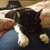New Contributor II

Hello,

I have created a model which iterates through some feature layers, makes a select by location, and exports the selected features into a folder as shapefiles.

I have also created a second model which is in theory supposed to run the model above, and then iterate through the the shapefiles and add a new field to each featureclass.

At present, my model does not run the former model, and I do not understand how to edit the python code so that I can run both models sequentially.

I have attached screenshots of the former model (Model4) and latter model (Model3), and the python code exported from the latter model.

Model4: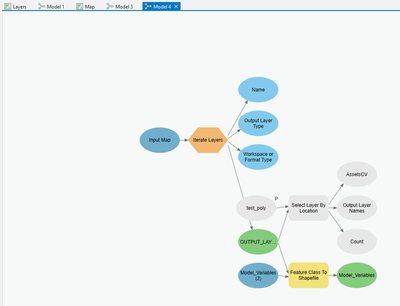Model3: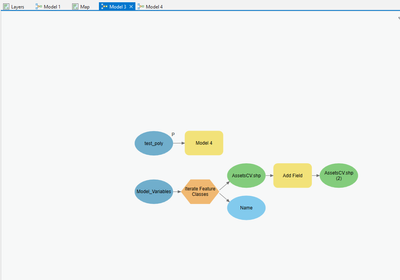Python Export:

``````# -*- coding: utf-8 -*-
"""
Generated by ArcGIS ModelBuilder on : 2022-05-10 18:24:07
"""
import arcpy
import os

from Default.Model4 import Model4
from sys import argv
def FeatureClassGenerator(workspace, wild_card, feature_type, recursive) :
with arcpy.EnvManager(workspace = workspace):

dataset_list = [""]
if recursive:
datasets = arcpy.ListDatasets()
dataset_list.extend(datasets)

for dataset in dataset_list:
featureclasses = arcpy.ListFeatureClasses(wild_card, feature_type, dataset)
for fc in featureclasses:
yield os.path.join(workspace, dataset, fc), fc

def Model3(test_poly="test_poly"): # Model 3

# To allow overwriting outputs change overwriteOutput option to True.
arcpy.env.overwriteOutput = False

Model_Variables = "C:\\Users\\Scar\\Documents\\landCharges\\landCharges\\Model_Variables"

for AssetsCV_shp, Name in FeatureClassGenerator(Model_Variables, "", "", "NOT_RECURSIVE"):

AssetsCV_shp_2_ = arcpy.management.AddField(in_table=AssetsCV_shp, field_name="Reference", field_type="TEXT", field_precision=None, field_scale=None, field_length=100, field_alias="", field_is_nullable="NULLABLE", field_is_required="NON_REQUIRED", field_domain="")

# Process: Model 4 (Model 4) (Default)
Model4(test_poly=test_poly)

if __name__ == '__main__':
# Global Environment settings
with arcpy.EnvManager(outputCoordinateSystem="PROJCS["British_National_Grid",GEOGCS["GCS_OSGB_1936",DATUM["D_OSGB_1936",SPHEROID["Airy_1830",6377563.396,299.3249646]],PRIMEM["Greenwich",0.0],UNIT["Degree",0.0174532925199433]],PROJECTION["Transverse_Mercator"],PARAMETER["False_Easting",400000.0],PARAMETER["False_Northing",-100000.0],PARAMETER["Central_Meridian",-2.0],PARAMETER["Scale_Factor",0.9996012717],PARAMETER["Latitude_Of_Origin",49.0],UNIT["Meter",1.0]]", scratchWorkspace=r"C:\Users\Scar\Documents\landCharges\landCharges\Default.gdb", workspace=r"C:\Users\Scar\Documents\landCharges\landCharges\Default.gdb"):
Model3(*argv[1:])``````

From what I understand from the python code, Model4 is being imported, but is not being called.I've had a look at the documentation for arcpy.EnvManager, but I haven't found any info on how an imported model is called as an argument within another.

Any help is much appreciated.

Tags (3)
1 Solution

Accepted Solutions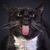Occasional Contributor III

Here's the thing about iterators in Model Builder.  They run everything in the model, regardless of where that tool or sub model is in relations to the Iterator.   For example, my brain would tell me that since model 4 is running before the iterator is fired in Model 3, Model 4 would run once and then Model 3's iterator would loop as many times as necessary, but that is not the case.  In your model 3, each time that iterator loops Model 4's iterator will loop.

One way to deal with this is don't use a sub model containing an iterator as an input into another model that contains an iterator.  For example; have a third "Main" model that calls Model 4 and then calls Model 3 rather than calling Model 4 directly from model 3.

6 RepliesbyMVP Frequent Contributor

Can just select stuff in one model, copy, paste into the other and connect them.

Is there specific reason you need two different models.

Also, more likely to get responses with code question if you use the code editor to insert code into the post.  Otherwise, it loses all indents and structure, and becomes hard to follow.

R_New Contributor II

Also, more likely to get responses with code question if you use the code editor to insert code into the post.

- I couldn't see the code editor first time around but that looks much more accessible now, thanks!

Can just select stuff in one model, copy, paste into the other and connect them.

- When I'm in the model builder and I can't directly connect Model4 to any process as it isn't a variable. For reference, it doesn't seem to me that the function from Model4 is being used in the code for Model3. I'm not sure how I can signal to Model Builder that in essence I would like the function from Model4 to run prior to the function created for Model3.

This is perhaps due to my lack of knowledge of how the arcpy.EnvManger parameters work - if anyone has any tips on that it would be appreciated.

Model3:

``````# -*- coding: utf-8 -*-
"""
Generated by ArcGIS ModelBuilder on : 2022-05-11 12:46:03
"""
import arcpy
import os

from Default.Model4 import Model4
from sys import argv
def FeatureClassGenerator(workspace, wild_card, feature_type, recursive) :
with arcpy.EnvManager(workspace = workspace):

dataset_list = [""]
if recursive:
datasets = arcpy.ListDatasets()
dataset_list.extend(datasets)

for dataset in dataset_list:
featureclasses = arcpy.ListFeatureClasses(wild_card, feature_type, dataset)
for fc in featureclasses:
yield os.path.join(workspace, dataset, fc), fc

def Model3(test_poly="test_poly"):  # Model 3

# To allow overwriting outputs change overwriteOutput option to True.
arcpy.env.overwriteOutput = False

Model_Variables = "C:\\Users\\Scar\\Documents\\landCharges\\landCharges\\Model_Variables"

for AssetsCV_shp, Name in FeatureClassGenerator(Model_Variables, "", "", "NOT_RECURSIVE"):

AssetsCV_shp_2_ = arcpy.management.AddField(in_table=AssetsCV_shp, field_name="Reference", field_type="TEXT", field_precision=None, field_scale=None, field_length=100, field_alias="", field_is_nullable="NULLABLE", field_is_required="NON_REQUIRED", field_domain="")

# Process: Model 4 (Model 4) (Default)
Model4(test_poly=test_poly)

if __name__ == '__main__':
# Global Environment settings
with arcpy.EnvManager(outputCoordinateSystem="PROJCS["British_National_Grid",GEOGCS["GCS_OSGB_1936",DATUM["D_OSGB_1936",SPHEROID["Airy_1830",6377563.396,299.3249646]],PRIMEM["Greenwich",0.0],UNIT["Degree",0.0174532925199433]],PROJECTION["Transverse_Mercator"],PARAMETER["False_Easting",400000.0],PARAMETER["False_Northing",-100000.0],PARAMETER["Central_Meridian",-2.0],PARAMETER["Scale_Factor",0.9996012717],PARAMETER["Latitude_Of_Origin",49.0],UNIT["Meter",1.0]]", scratchWorkspace=r"C:\Users\Scar\Documents\landCharges\landCharges\Default.gdb", workspace=r"C:\Users\Scar\Documents\landCharges\landCharges\Default.gdb"):
Model3(*argv[1:])``````

Model4:

``````# -*- coding: utf-8 -*-
"""
Generated by ArcGIS ModelBuilder on : 2022-05-11 12:46:26
"""
import arcpy
from sys import argv
def #  NOT  IMPLEMENTED# Function Body not implemented

def Model4(test_poly):  # Model 4

# To allow overwriting outputs change overwriteOutput option to True.
arcpy.env.overwriteOutput = False

arcpy.ImportToolbox(r"c:\program files\arcgis\pro\Resources\ArcToolbox\toolboxes\Conversion Tools.tbx.tbx")
Input_Map = "Layers"
Model_Variables_2_ = "C:\\Users\\Scar\\Documents\\landCharges\\landCharges\\Model_Variables"

for OUTPUT_LAYER, Name, Output_Layer_Type, Workspace_or_Format_Type in #  NOT  IMPLEMENTED(Input_Map, "", ["FeatureLayer"], [], [], [], "ALL", "ALL", "RECURSIVE"):

# Process: Select Layer By Location (Select Layer By Location) (management)
AssetsCV, Output_Layer_Names, Count = arcpy.management.SelectLayerByLocation(in_layer=[OUTPUT_LAYER], overlap_type="INTERSECT", select_features=test_poly, search_distance="", selection_type="NEW_SELECTION", invert_spatial_relationship="NOT_INVERT")

# Process: Feature Class To Shapefile (Feature Class To Shapefile) (conversion)
Model_Variables = arcpy.conversion.FeatureClassToShapefile(Input_Features=[OUTPUT_LAYER], Output_Folder=Model_Variables_2_)

if __name__ == '__main__':
# Global Environment settings
with arcpy.EnvManager(outputCoordinateSystem="PROJCS["British_National_Grid",GEOGCS["GCS_OSGB_1936",DATUM["D_OSGB_1936",SPHEROID["Airy_1830",6377563.396,299.3249646]],PRIMEM["Greenwich",0.0],UNIT["Degree",0.0174532925199433]],PROJECTION["Transverse_Mercator"],PARAMETER["False_Easting",400000.0],PARAMETER["False_Northing",-100000.0],PARAMETER["Central_Meridian",-2.0],PARAMETER["Scale_Factor",0.9996012717],PARAMETER["Latitude_Of_Origin",49.0],UNIT["Meter",1.0]]", scratchWorkspace=r"C:\Users\Scar\Documents\landCharges\landCharges\Default.gdb", workspace=r"C:\Users\Scar\Documents\landCharges\landCharges\Default.gdb"):
Model4(*argv[1:])``````

Thanks for taking a look!Occasional Contributor III

Looks like Model 4 did not validate.  That probably indicates an error in Model 4.

I'm guessing the OUTPUT LAYER parameter from Iterate Layers in Model 4 should not be used as input into the Feature Class To Shapefile tool.  Rather the output of the Select Layer By Location tool should feed the Feature Class to Shapefile tool in Model 4.  Something like this: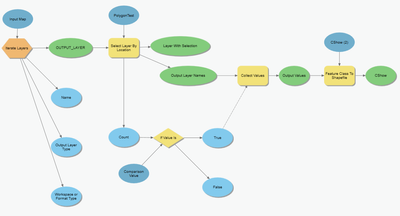Also, I agree with @RhettZufelt.  I don't see why two models are necessary.  You can add the attributes to the shapefiles as they are created, but if you do want to create the shapefiles first and add the fields later you will need 2 models (since their is a limit of one iterator per model as you know).... To use two model try making Model 4 a precondition of Model 3.New Contributor II

That's very helpful thank you! I've revalidated** Model4 (for ref:)and re-added the new validated model to Model3 which is now adding it to the body of Model3's main function (for ref:)

``````# -*- coding: utf-8 -*-
"""
Generated by ArcGIS ModelBuilder on : 2022-05-11 15:35:05
"""
import arcpy
import os

from Default.Model4 import Model4
def FeatureClassGenerator(workspace, wild_card, feature_type, recursive) :
with arcpy.EnvManager(workspace = workspace):

dataset_list = [""]
if recursive:
datasets = arcpy.ListDatasets()
dataset_list.extend(datasets)

for dataset in dataset_list:
featureclasses = arcpy.ListFeatureClasses(wild_card, feature_type, dataset)
for fc in featureclasses:
yield os.path.join(workspace, dataset, fc), fc

def Model3():  # Model 3

# To allow overwriting outputs change overwriteOutput option to True.
arcpy.env.overwriteOutput = False

Model_Variables = "C:\\Users\\Scar\\Documents\\landCharges\\landCharges\\Model_Variables"
test_poly = "test_poly"

for AssetsCV_shp, Name in FeatureClassGenerator(Model_Variables, "", "", "NOT_RECURSIVE"):

AssetsCV_shp_2_ = arcpy.management.AddField(in_table=AssetsCV_shp, field_name="Reference", field_type="TEXT", field_precision=None, field_scale=None, field_length=100, field_alias="", field_is_nullable="NULLABLE", field_is_required="NON_REQUIRED", field_domain="")

# Process: Model 4 (Model 4) (Default)
Model4(test_poly=test_poly)

if __name__ == '__main__':
# Global Environment settings
with arcpy.EnvManager(outputCoordinateSystem="PROJCS["British_National_Grid",GEOGCS["GCS_OSGB_1936",DATUM["D_OSGB_1936",SPHEROID["Airy_1830",6377563.396,299.3249646]],PRIMEM["Greenwich",0.0],UNIT["Degree",0.0174532925199433]],PROJECTION["Transverse_Mercator"],PARAMETER["False_Easting",400000.0],PARAMETER["False_Northing",-100000.0],PARAMETER["Central_Meridian",-2.0],PARAMETER["Scale_Factor",0.9996012717],PARAMETER["Latitude_Of_Origin",49.0],UNIT["Meter",1.0]]", scratchWorkspace=r"C:\Users\Scar\Documents\landCharges\landCharges\Default.gdb", workspace=r"C:\Users\Scar\Documents\landCharges\landCharges\Default.gdb"):
Model3()``````

I can see that Model 4 is being run recursively in the main body of Model3, making the processes very lengthy. I'm unsure if there is a way to alter the code of the Model directly, but I would like Model4 to run before the for loop in the main body of the Model3 function.

After I run these processes, I would like to iterate through the created shapefiles and export their tables to a .csv file, and then colate these .csv's into a single file.

**For ref on the validation in case anyone is having similar trouble: My model was running correctly despite not being validated if you ran it as a geoprocessing tool. I was able to fix the validation by creating a new variable in model builder and setting it as a feature layer, and selecting the 'test_poly' as the default feature layer for when the model is run in model builder as opposed to a geoprocessing tool. The problem here was that the geoprocessing tool prompted the user for a variable it would use for the process, whereas the model had no such prompt.Occasional Contributor III

Here's the thing about iterators in Model Builder.  They run everything in the model, regardless of where that tool or sub model is in relations to the Iterator.   For example, my brain would tell me that since model 4 is running before the iterator is fired in Model 3, Model 4 would run once and then Model 3's iterator would loop as many times as necessary, but that is not the case.  In your model 3, each time that iterator loops Model 4's iterator will loop.

One way to deal with this is don't use a sub model containing an iterator as an input into another model that contains an iterator.  For example; have a third "Main" model that calls Model 4 and then calls Model 3 rather than calling Model 4 directly from model 3.New Contributor II

That's done the trick! I've taken Model4 out of Model3 and run both Models in a main Model instead.

That's excellent info, I couldn't find anything similar on the Esri documentation. Now I can move on to trying to collate all of the tables into one export - thanks again!